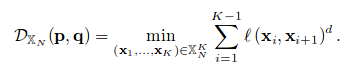library to compute fermat distance

# Fermat distance

Fermat is a Python library that computes the Fermat distance estimator (also called d-distance estimator) proposed in

### Introduction

A density-based estimator for weighted geodesic distances is proposed. Let M be a D-dimensional manifold and consider a sample of N points X_n living in M. Let l(.,.) be a distance defined in M (a typical choice could be Euclidean distance). For d>=1 and given two points p and q in M we define the Fermat distance estimator asThe minimization is done over all K>=2 and all finite sequences of data points with x1= argmin l(x,p) and xK = argmin l(x,q).

When d=1, we recover the distance l(.,.) but if d>1, the Fermat distance tends to follow more closely the manifold structure and regions with high density values.

This distance is useful for clustering pourposes. Replacing Euclidean distance with Fermat distance in standard clustering alorithms leads to much better results.## Installation

pip install fermat


### Implementation

The optimization performed to compute the Fermat distance estimator runs all over the possible paths of points between each pair of points. We implement an algorithm that computes the exact Fermat distance and two that compute approximations.

• #### Exact: Floyd-Warshall

Permorf the Floyd-Warshall algorithm that gives the exact Fermat distance estimator in O( n^3 ) operations between all possible paths that conects each pair of points.

• #### Aprox: Dijsktra + k-nearest neighbours

With probability arbitrary high we can restrict the minimum path search to paths where each consecutive pair of points are k-nearest neighbours, with k = O(log n). Then, we use Dijkstra algorithm on the graph of k-nearest neighbours from each point. The complexity is O( n * ( k * n * log n ) ).

• #### Aprox: Landmarks

If the number of points n is too high and neither Floyd-Warshall and Dijkstra run in appropiate times, we implemente a gready version based on landmarks. Let consider a set of l of point in the data set (the landmarks) and denote s_j the distance of the point s to the landmark j. Then, we can bound the distance d(s,t) between any two points s and t as

lower = max_j { | s_j - t_j | } <= d(s,t) <= min_j { s_j + t_j } = upper

and estimate d(s,t) as a function of lower and upper (for example, d(s,t) ~ (_lower + upper_) / 2 ). The complexity is O( l * ( k * n * log n ) ).

### Clustering

The tool FermatKmeans implements the K-medoids algorithm with Fermat distance as an input. Here is an example explaining how to use it to compute clusters in MNIST dataset

### Support

If you have an open-ended or a research question:

• 'support@aristas.com.ar'

### Fermat distance citation

Fermat distance has been introduced and studied in the following papers

@inproceedings{SGJ2018,
title={Weighted Geodesic Distance Following Fermat's Principle},
author={Facundo Sapienza and Pablo Groisman and Matthieu Jonckheere},
year={2018},
url={https://openreview.net/forum?id=BJfaMIJwG}
}

@article{GJS2018,
title={Nonhomogeneous Euclidean first-passage percolation and distance learning},
author={Groisman, Pablo and Jonckheere, Matthieu and Sapienza, Facundo},
journal={arXiv preprint arXiv:1810.09398},
year={2018}
}


## Project details

This version0.2.70.2.50.2.40.2.20.2.00.1.00.0.3

Uploaded py3